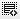## Assuming a 15% risk-free rate, cost of equity of 25% and a beta of 1, what is the excess market premium?00

Kce=RFR + Beta(EMP-RFR)
=>By putting the values EMP= 25%0

Dipanjan is right. That is the correct formula. Once you substitute you will get it.000

Sorry the answer is not 25 %.. sir can u eloborate o this pls..

Assuming a 15% risk-free rate, cost of equity of 25% and a beta of 1, what is the excess market premium?

10% - Correct
15%
25% - Incorrect

Click on thisicon to add code snippet.